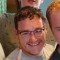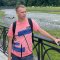# Getting started with PHP in MODXLast updated Aug 27th, 2019 | Page history | Improve this page | Report an issue

So, a lot of people have been asking about the new codebase. Is it coder-friendly? Will it be a big deviation from 0.9.6/Evolution? Does it support OOP projects? Is it faster? Will it be easy to learn?

In these tutorials, we plan to answer those questions with YES.

The codebase in Revolution has switched to xPDO, an object relational bridge modeling tool built by Jason Coward. In layman's terms, this means that all the database tables are now represented by PHP objects (as is common with any ORM). Chunks are represented by 'modChunk' objects, snippets by 'modSnippet' objects and so on.

## The Simple How¶

So, how does one actually get an object in the new modx? Well, you used to have to rely on a handful of different functions:

``````// The old way of doing things in MODx 1.x and earlier
\$doc = \$modx->getDocument(23);
\$doc = \$modx->getDocument(45,'pagetitle,introtext');
\$chunk = \$modx->getChunk('chunkName');

// or even more convoluted
\$users = array();
if (\$modx->db->getRecordCount(\$res))
{
while (\$row = \$modx->db->getRow(\$res)) {
array_push(\$users,\$row);
}
}
return \$users;
``````

Not anymore. Things are much simpler, and there's really only a few functions you'll need. Lets look at some examples:

``````// getting a chunk with ID 43
\$chunk = \$modx->getObject('modChunk',43);

// getting a chunk with name 'TestChunk'
\$chunk = \$modx->getObject('modChunk',array(
'name' => 'TestChunk'
));

// getting a collection of chunk objects, then outputting their names
\$chunks = \$modx->getCollection('modChunk');
foreach (\$chunks as \$chunk) {
echo \$chunk->get('name')."<br />\n";
}

// getting a resource (i.e. a page) that is published, with a alias of 'test'
\$document = \$modx->getObject('modResource',array(
'published' => 1,
'alias' => 'test',
));
``````

## The Model¶

So, you're probably asking, Where is the list of table names to object names map? It can be found in "core/model/schema/modx.mysql.schema.xml". (You'll note the 'mysql' - yes, this means that MODX will in the near future support other databases) From there you can view an XML representation of all the MODX DB tables.

For example, modChunk:

``````<object class="modChunk" table="site_htmlsnippets" extends="modElement">
<field key="name" dbtype="varchar" precision="50" phptype="string" null="false" default="" index="unique" />
<field key="description" dbtype="varchar" precision="255" phptype="string" null="false" default="Chunk" />
<field key="editor_type" dbtype="int" precision="11" phptype="integer" null="false" default="0" />
<field key="category" dbtype="int" precision="11" phptype="integer" null="false" default="0" />
<field key="cache_type" dbtype="tinyint" precision="1" phptype="integer" null="false" default="0" />
<field key="snippet" dbtype="mediumtext" phptype="string" />
<field key="locked" dbtype="tinyint" precision="1" attributes="unsigned" phptype="boolean" null="false" default="0" />
<aggregate alias="Category" class="modCategory" key="id" local="category" foreign="id" cardinality="one" owner="foreign" />
</object>
``````

You can also define your own schemas for your own components and add them as packages - more on that in a future article. Lets go into the schema:

``````<object class="modChunk" table="site_htmlsnippets" extends="modElement">
``````

The class property tells you what the name of the class will be. The table property shows the actual MySQL table, and extends shows what object it extends. modElement is a base class for all Elements in MODX - snippets, modules, chunks, templates, etc.

``````<field key="name" dbtype="varchar" precision="50" phptype="string" null="false" default="" index="unique" />
``````

This tag represents a column in the database. Most of these attributes are pretty straightforward.

``````<aggregate alias="modCategory" class="modCategory" key="id" local="category" foreign="id" cardinality="one" owner="foreign" />
``````

Okay, this is where we get into DB relationships. An Aggregate relationship is a relationship where, in laymans terms, if you were to delete this chunk, it wouldn't delete the Category that it's related to. If it were a Composite relationship, it would. There is "dependence" in the Composite relationship that is related to the other object. For an example, let's get all the modContextSettings for a modContext:

``````\$context = \$modx->getObject('modContext','web');
\$settings = \$context->getMany('ContextSetting');
foreach (\$settings as \$setting) {
echo 'Setting name: '.\$setting->get('key').' <br />';
echo 'Setting value: '.\$setting->get('value').' <br />';
}
``````

Pretty easy, huh? We'll get into creating and removing objects, as well as more complex queries, such as inner joins, limits, sorting and others, in the next article.